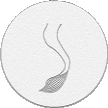## Sunday, March 3, 2013

### Balloon starMaterials: a balloon; an air pump and a scissors.

We are going to make a five points star with a stick at the center of the star. We can call it a five petals flower as well. Inflate a balloon leaving a five—inch flat tail (Pic 1).
Twist the first two—inch bubble (Pic 2).
Twist the second two—inch bubble (Pic 3).
Lock both ends of the chain of the first two (the first and the second) bubbles in one lock twist (Pic 4). This step is marked with arrows between points A and B on the scheme.
Twist the third two—inch bubble (Pic 5).
Twist the fourth two—inch bubble (Pic 6).
Lock both ends of the chain of the last two (the third and the fourth) bubbles in one lock twist (Pic 7). This step is marked with arrows between points B and C on the scheme.
Twist the fifth two—inch bubble (Pic 8).
Twist the sixth two—inch bubble (Pic 9).
Lock both ends of the chain of the last two (the fifth and the sixth) bubbles in one lock twist (Pic 10). This step is marked with arrows between points C and D on the scheme.
Twist the seventh two—inch bubble (Pic 11).
Twist the eighth two—inch bubble (Pic 12).
Lock both ends of the chain of the last two (the seventh and the eighth) bubbles in one lock twist (Pic 13). This step is marked with arrows between points D and E on the scheme.
Twist the ninth two—inch bubble (Pic 14).
Twist the tenth two—inch bubble (Pic 15).
Lock both ends of the chain of the last two (the ninth and the tenth) bubbles in one lock twist (Pic 16).This step is marked with arrows between points E and F on the scheme. The rest of the balloon is the eleventh bubble.
You can cut off the eleventh bubble and tie off the end (Pic 17).
You can use it as a stick to hold the star or as a stem for the flower (Pic 18). Congratulations, you have made the five points star.
There is an example of how you can attach the star to the Christmas tree (Pic 19 and 20). Have fun and happy twisting :)
So far you spent on this page.

www.fewdoit.com
for you